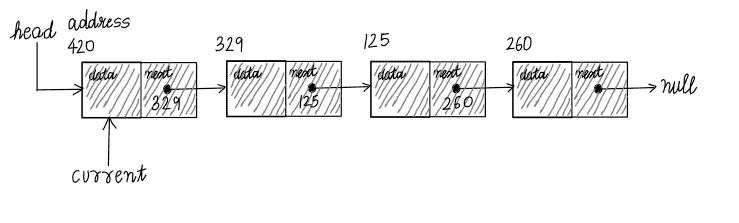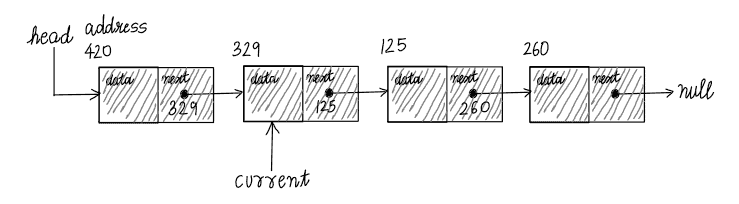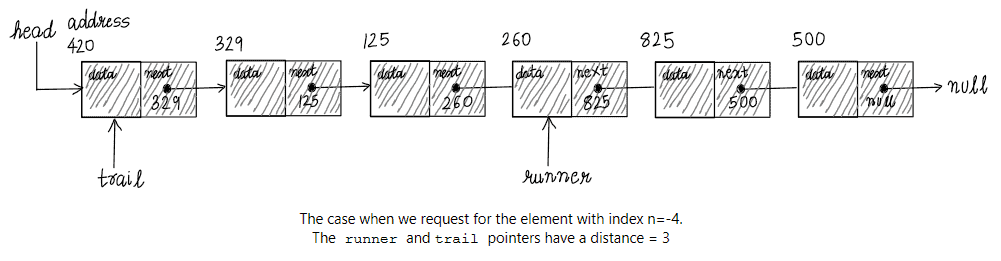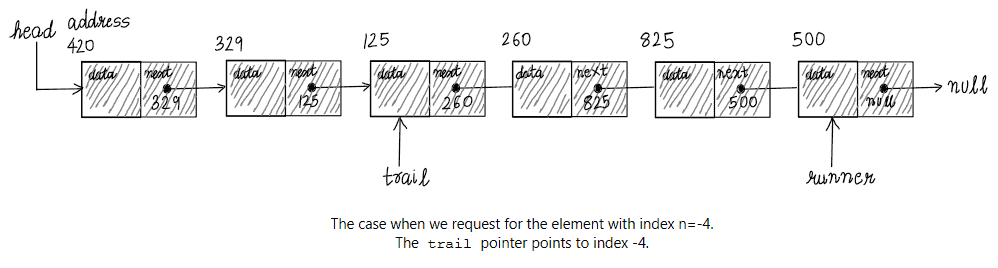## 1. Introduction

The singly linked list is a common data structure implemented in most high-level programming languages. For instance, these are implementations of singly linked lists:

• C++ STL type std::foward_list
• Scala and F# type List

In its most basic form, a linked list consists of a collection of nodes. Each node contains data and a reference to the next node.

In this tutorial, we’ll learn how to find theth element of the singly linked list by traversing through the list.

## 2. Algorithm to Find the nth Element

We are interested in implementing a function that gets theth element of a linked list. How might we write such a function? To get theth element, we must traverse through the list.

We introduce an algorithm for getting the element with index,of a singly linked list. In the subsequent section, we shall go over each of the important steps in a structured manner.

Consider the following pseudo-code:## 3. A Walk-Through of GetItem(Head,n)

Our initial setup is as follows:We take an empty pointer current and set it to refer to the head node – thest node of the linked list. current always refers to our current position in the linked list.

current.next points to the successive node – thend node of the linked list. If we assign current = current.next, this will move current to the right. The below snap is just after current is moved to the right:Executing current = current.next multiple times in a while loop allows us to traverse through the linked list. However, we must be careful not to go beyond the end of the linked list. If current = null, invoking current = current.next on a null object will cause our program to crash. So, we write the boundary condition current!=null to control the maximum number of iterations.

The logic so far helps us move through the list. However, it would be good to keep a track of the index we are at. Hence, we use a counter-variable i and count to n. First, we initialize i=0, and inside each iteration, we check if the counter i equals n. If this condition is true, we return the data inside the current node to the caller function. If false, we proceed with the next iteration, moving current to the next successive node and increasing the value of the counter variable i by.

If the control reaches the statement following the end of the while-loop, it implies that we have reached the end of the list before counting to n.

## 4. Algorithm to Find the nth Element From the Tail of the List

We can build upon the above basic technique to find theth element from the end of the singly linked list. To be consistent, we use the following convention. We shall refer to the tail of the linked list as the element having index, the last but one element as having index, and so forth.

We are interested in writing a function that takes the head of the linked list as the first argument and an index () as the second argument.

Consider the following pseudo-code for getting the element with index, whereof a linked list:## 5. A Walk-Through of GetItemFromEnd(head,n)

Let’s walk through the important steps of the algorithm introduced in the previous section. We take an empty pointer runner and initialize it to refer to the head node of the linked list. We now traverse through the list, moving runner to the node with index.

At this point, we may have already reached the end of the linked list, in which case runner = null. In such a situation, we abort and print a message to the user that the list is too small. If this is not the case, we take an empty pointer trail and set it to refer to the head node of the list.

The distance between the runner and trail pointers is equal to. So, our setup is as follows:Now, we move both – the runner and the trail pointers to their respective successive nodes iteratively. Throughout these iterations, the distancebetween the runner and the trail is kept constant. Thus, when runner refers to the tail of the linked list, the trail pointer should point to theth node from the end of the list.

The below snap depicts the situation, when runner has reached the end of the list, for the case:## 6. Conclusion

In this article, we learned about the algorithms to get an element with indexof a linked list. To recap, first, retrieving an element from the beginning of the list requires initializing a current pointer to head. It should then be moved to its successor nodetimes. Second, when retrieving an element from the end of the list, we set up two pointers runner and trail, a distanceapart. We then move both pointers iteratively to their successor nodes until runner.next=null.